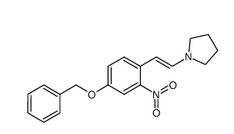## Diss FactsheetsPhysical & Chemical properties

## Vapour pressure

Endpoint:
vapour pressure
Type of information:
experimental study
key study
Study period:
15/05/2017 - 07/07/2017
Reliability:
1 (reliable without restriction)
Rationale for reliability incl. deficiencies:
guideline study

Reference Type:
study report
Title:
Unnamed
Year:
2018
Report date:
2018

### Materials and methods

##### Test guideline
Qualifier:
according to guideline
Guideline:
OECD Guideline 104 (Vapour Pressure Curve)
Version / remarks:
Deviations:
no
Qualifier:
according to guideline
Guideline:
EU Method A.4 (Vapour Pressure)
Version / remarks:
24/08/2009
Deviations:
no
GLP compliance:
yes (incl. QA statement)
Type of method:
effusion method: Knudsen cell

#### Test material

##### Constituent 1Reference substance name:
Pyrrolidine, 1-[2-[2-nitro-4-(phenylmethoxy)phenyl]ethenyl]-
Cas Number:
99474-22-3
Molecular formula:
C19H20N2O3
IUPAC Name:
Pyrrolidine, 1-[2-[2-nitro-4-(phenylmethoxy)phenyl]ethenyl]-
Specific details on test material used for the study:
- Analytical purity: > 98.4%
- Lot/batch No.: 0159115
- Expiration date of the lot/batch: 21/02/2018

### Results and discussion

##### Vapour pressure
Key result
Temp.:
ca. 20 °C
Vapour pressure:
ca. 0 Pa
Remarks on result:
other: calculated from the regression equation
Key result
Temp.:
ca. 25 °C
Vapour pressure:
ca. 0 Pa
Remarks on result:
other: Calculated from the regression equation
Temp.:
ca. 75 °C
Vapour pressure:
ca. 0 Pa
Remarks on result:
other: experimental result
Temp.:
ca. 90 °C
Vapour pressure:
ca. 0.002 Pa
Remarks on result:
other: experimental result
Temp.:
ca. 105 °C
Vapour pressure:
ca. 0.012 Pa
Remarks on result:
other: experimental value
##### Transition / decomposition
Key result
Transition / decomposition:
no

## Observations

75°C. At the first measurement of experiment 4, all cells were slightly contaminated. Therefore, the cells were meticulously cleaned and weighed. This weight was used as initial weight for the subsequent measurements. To avoid bias, this measurement was not used for calculations.

90°C. At the second measurement of experiment 5, all cells showed no weight loss. On the basis of the first and third measurement the second measurement was defined as outlier. To avoid bias, this measurement was not used for calculations.

105°C. At the first, second and third measurement of experiment 6, cell 1 and 3 were slightly contaminated. Therefore, the cells were meticulously cleaned and weighed. This weight was used as initial weight for the subsequent measurements. To avoid bias, this measurement was not used for calculations.

150°C. At the first measurement of experiment 9, all cells were empty.

## Evaluation

The measured values for temperature and vapour pressure were evaluated as follows:

 Parameter T Mean P 1/T log P Unit K Pa 1/K log Pa Values 348.2 4.55E-04 2.8719E-03 -3.3415 363.2 2.46E-03 2.7533E-03 -2.6095 378.2 1.19E-02 2.6441E-03 -1.9239

A graph with log P vs. 1/T was plotted. Since the values were correlated by a linear relation, the data could be fitted by linear regression.

Parameters of Linear Regression

 Parameter Value Slope -6222.3 Intercept 14.5265 CorrelationCoefficient r -1.0000 Coefficient ofDeterminationr2 1.0000

# Results

## Test Item

The following vapour pressures were determined experimentally and considered as valid (mean and standard deviation given):

Experimentally Determined Vapour Pressures

 T T P Stand. Dev. P RSD P °C K Pa Pa % 75.0 348.2 4.55E-04 9.47E-05 20.8% 90.0 363.2 2.46E-03 4.76E-04 19.4% 105.0 378.2 1.19E-02 2.83E-03 23.8%

Stand. Dev. = Standard Deviation; RSD = Relative Standard Deviation

For the test item, the following vapour pressures at 20°C and at 25°C were calculated from the regression equation:

 Vapour pressure at 20°C: 2.00* 10-07Pa Vapour pressure at 25°C: 4.54* 10-07Pa

The linear regression of log P vs. 1/T gave a correlation coefficient r of - 1.0000, showing good repeatability and precision. Therefore, the determination is considered as valid.

## Positive Control

 Vapour pressure (positive control) at 30°C: 2.03*10-1Pa Criteria for thevapourpressure of the positive control at 30°C 1.44*10-1± 0.1Pa

The vapour pressure met the validity criteria. Therefore the study can be considered as valid.

### Applicant's summary and conclusion

Conclusions:
For the test item, the following vapour pressures at 20 °C and at 25 °C were calculated from the regression equation:
-       Vapour pressure at 20°C: 2.00E-07 Pa
-       Vapour pressure at 25°C: 4.54E-07 Pa
Executive summary:

The vapour pressure of the test item was determined according to OECD 104 resp. EU A.4 using the effusion method (weight loss; GLP study).

Vapour pressure was examined ateight different temperatures. Three temperatures could be evaluated as they showed reproducible weight loss. For these temperatures, 1/T was plotted against log (P).

 Experiment T MeanP Stand. Dev. P RSD P No. K Pa Pa % 4 348.2 4.55E-04 9.47E-05 20.8% 5 363.2 2.46E-03 4.76E-04 19.4% 6 378.2 1.19E-02 2.83E-03 23.8%

Stand. Dev. = Standard Deviation; RSD = Relative Standard Deviation

The graph 1/T vs. log (P) shows a straight line with a correlation coefficient of - 1.0000.

For the test item, the following vapour pressures at 20 °C and at 25 °C were calculated from the regression equation:

-       Vapour pressure at 20°C: 2.00E-07 Pa

-       Vapour pressure at 25°C: 4.54E-07 Pa